Opencv中Mat矩阵相乘——点乘、dot、mul运算详解

# Opencv中Mat矩阵相乘——点乘、dot、mul运算详解

2016年09月02日 00:00:36 -牧野- 阅读数：59593 标签： Opencv矩阵相乘点乘dotmul 更多

# Mat矩阵点乘——A*B

Opencv重载了运算符“*”，姑且称之为Mat矩阵“点乘”，其中一个重载声明为：

``````CV_EXPORTS MatExpr operator * (const Mat& a, const Mat& b);
``````

## 点乘说明：

1.  A*B是以数学运算中矩阵相乘的方式实现的，即Mat矩阵A和B被当做纯粹的矩阵做乘法运算，这就要求A的列数等       于B的行数时，才能定义两个矩阵相乘。如A是m×n矩阵，B是n×p矩阵，它们的乘积AB是一个m×p矩阵。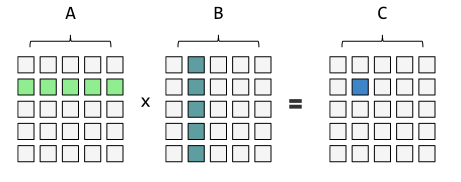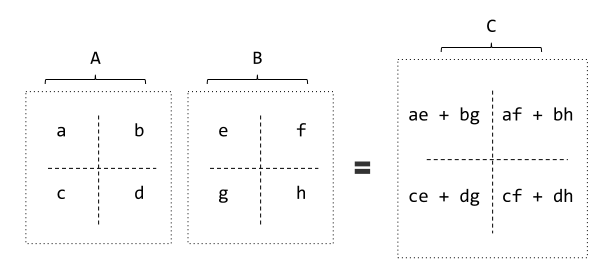Opencv验证：

1. #include "core/core.hpp"

2. #include "iostream"

3. using namespace std;

4. using namespace cv;

5. int main(int argc,char *argv[])

6. {

7. Mat A=Mat::ones( 2,3,CV_32FC1);

8. Mat B=Mat::ones( 3,2,CV_32FC1);

9. Mat AB;

10. A.at< float>(0,0)=1;

11. A.at< float>(0,1)=2;

12. A.at< float>(0,2)=3;

13. A.at< float>(1,0)=4;

14. A.at< float>(1,1)=5;

15. A.at< float>(1,2)=6;

16. B.at< float>(0,0)=1;

17. B.at< float>(0,1)=2;

18. B.at< float>(1,0)=3;

19. B.at< float>(1,1)=4;

20. B.at< float>(2,0)=5;

21. B.at< float>(2,1)=6;

22. AB=A*B;

23. cout<<"A=\n"<<A<<endl<<endl;

24. cout<<"B=\n"<<B<<endl<<endl;

25. cout<<"AB=\n"<<AB<<endl<<endl;

26. system( "pause");

27. }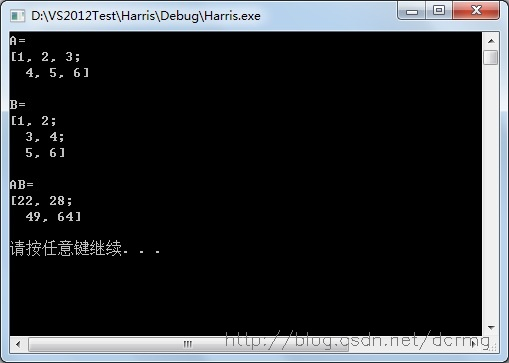2.  参与点乘的两个Mat矩阵的数据类型（type）只能是 CV_32F、 CV_64FC1、 CV_32FC2、 CV_64FC2 这4种类        型中的一种。若选用其他类型，比如CV_8UC1，编译器会报错：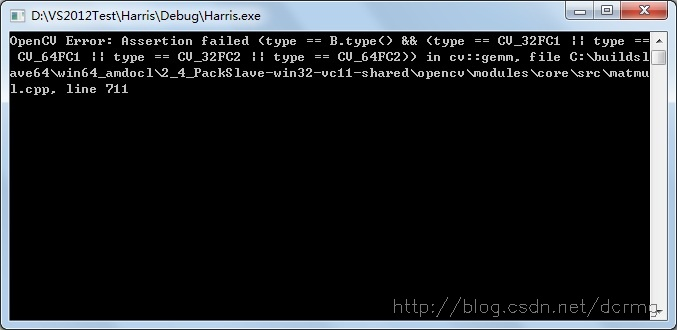# Mat矩阵dot——A.dot(B)

Opencv中.dot操作才算得上是真正的“点乘”，A.dot(B)操作相当于数学向量运算中的点乘，也叫向量的内积、数量积。

1. //! computes dot-product

2. double dot(InputArray m) const;

## dot说明：

1.  对两个向量执行点乘运算，就是对这两个向量对应位一一相乘之后求和的操作，点乘的结果是一个标量。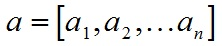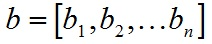a和b的点积公式为：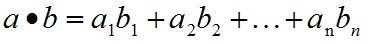Mat矩阵的dot方法扩展了一维向量的点乘操作，把整个Mat矩阵扩展成一个行（列）向量，之后执行向量的点乘运算，仍然要求参与dot运算的两个Mat矩阵的行列数完全一致。

2.  dot方法声明中显示返回值是double，所以A.dot(B)结果是一个double类型数据，不是Mat矩阵，不能把A.dot(B)结       果赋值给Mat矩阵！

Opencv验证：

1. #include "core/core.hpp"

2. #include "iostream"

3. using namespace std;

4. using namespace cv;

5. int main(int argc,char *argv[])

6. {

7. Mat A=Mat::ones( 2,3,CV_8UC1);

8. Mat B=Mat::ones( 2,3,CV_8UC1);

9. A.at( 0,0)=1;

10. A.at( 0,1)=2;

11. A.at( 0,2)=3;

12. A.at( 1,0)=4;

13. A.at( 1,1)=5;

14. A.at( 1,2)=6;

15. B.at( 0,0)=1;

16. B.at( 0,1)=2;

17. B.at( 0,2)=3;

18. B.at( 1,0)=4;

19. B.at( 1,1)=5;

20. B.at( 1,2)=6;

21. double AB=A.dot(B);

22. cout<<"A=\n"<<A<<endl<<endl;

23. cout<<"B=\n"<<B<<endl<<endl;

24. cout<<"double类型的AB=\n"<<AB<<endl<<endl;

25. system( "pause");

26. }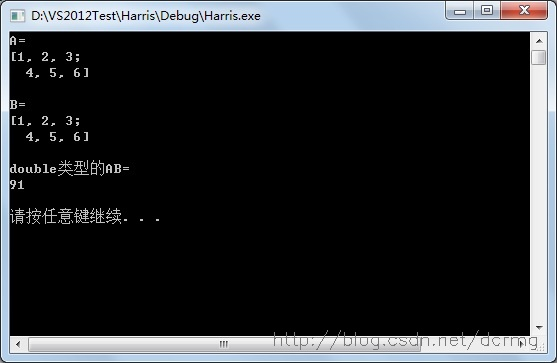3.  dot操作不对参与运算的矩阵A、B的数据类型做要求，CV_8UC1、CV_32FC1等，可以是任何Opencv定义的类         型，如在2中使用的就是CV_8UC1。

4.  若参与dot运算的两个Mat矩阵是多通道的，则计算结果是所有通道单独计算各自.dot之后，再累计的和，结果仍是一个double类型数据。

# Mat矩阵mul——A.mul(B)

Opencv中mul会计算两个Mat矩阵对应位的乘积，所以要求参与运算的矩阵A的行列和B的行列数一致。计算结果是跟A或B行列数一致的一个Mat矩阵。

Opencv中mul声明：

1. //! per-element matrix multiplication by means of matrix expressions

2. MatExpr mul(InputArray m, double scale=1) const;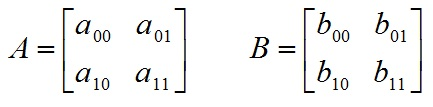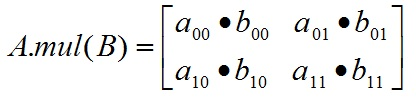## mul说明：

1.  mul操作不对参与运算的两个矩阵A、B有数据类型上的要求，但要求A，B类型一致，不然报错；

2.  Mat AB=A.mul(B)，若声明AB时没有定义AB的数据类型，则默认AB的数据类型跟A和B保存一致；

3.  若AB精度不够，可能产生溢出，溢出的值被置为当前精度下的最大值；

Opencv验证：

1. #include "core/core.hpp"

2. #include "iostream"

3. using namespace std;

4. using namespace cv;

5. int main(int argc,char *argv[])

6. {

7. Mat A=Mat::ones( 2,3,CV_8UC1);

8. Mat B=Mat::ones( 2,3,CV_8UC1);

9. A.at( 0,0)=60;

10. A.at( 0,1)=2;

11. A.at( 0,2)=3;

12. A.at( 1,0)=4;

13. A.at( 1,1)=5;

14. A.at( 1,2)=6;

15. B.at( 0,0)=60;

16. B.at( 0,1)=2;

17. B.at( 0,2)=3;

18. B.at( 1,0)=4;

19. B.at( 1,1)=5;

20. B.at( 1,2)=6;

21. Mat AB=A.mul(B);

22. cout<<"A=\n"<<A<<endl<<endl;

23. cout<<"B=\n"<<B<<endl<<endl;

24. cout<<"AB=\n"<<AB<<endl<<endl;

25. system( "pause");

26. }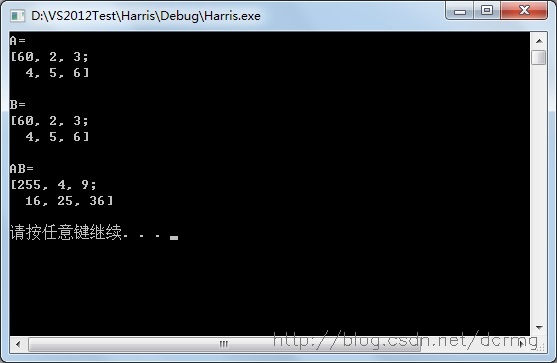AB中第一个元素应该为60*60=360，但AB默认的类型为CV_8UC1,即最大值只能是255；所以执行mul运算一定要定义AB足够的精度，防止溢出。刚刚好
4个月前
css问题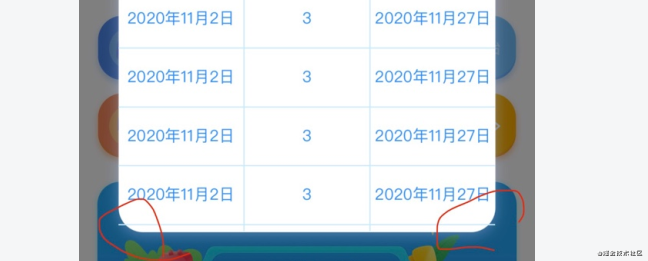blmius
1年前
MySQL:[Err] 1292 - Incorrect datetime value: ‘0000-00-00 00:00:00‘ for column ‘CREATE_TIME‘ at row 1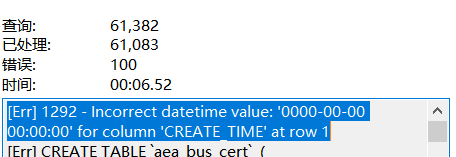小森森
4个月前晴空闲云
4个月前
css中box-sizing解放盒子实际宽高计算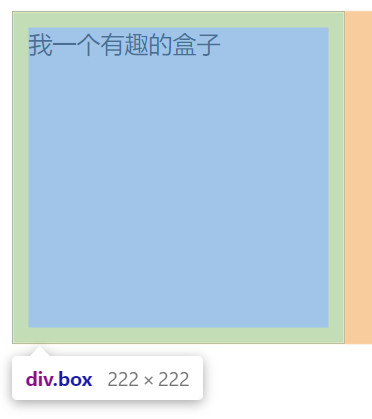艾木酱
3个月前

MemFireCloud是一款提供云数据库，用户可以创建云数据库，并对数据库进行管理，还可以对数据库进行备份操作。它还提供后端即服务，用户可以在1分钟内新建一个应用，使用自动生成的API和SDK，访问云数据库、对象存储、用户认证与授权等功能，可专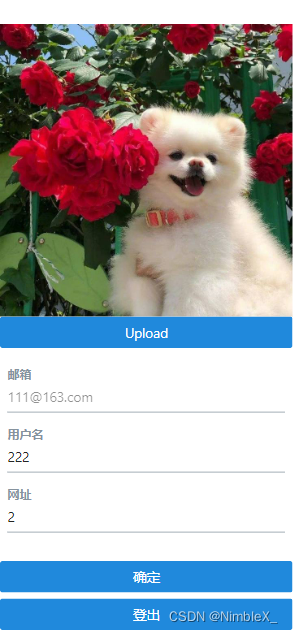Wesley13
1年前
Java爬虫之JSoup使用教程
title:Java爬虫之JSoup使用教程date:201812248:00:000800update:201812248:00:000800author:mecover:https://imgblog.csdnimg.cn/20181224144920712(https://www.oschinWesley13
1年前
Java日期时间API系列36
十二时辰，古代劳动人民把一昼夜划分成十二个时段，每一个时段叫一个时辰。二十四小时和十二时辰对照表：时辰时间24时制子时深夜11：00凌晨01：0023：0001：00丑时上午01：00上午03：0001：0003：00寅时上午03：00上午0Stella981
1年前
Angular material matWesley13
1年前
MySQL部分从库上面因为大量的临时表tmp_table造成慢查询helloworld_28799839
4个月前

Javascript判断对象是否为空jsObject.keys(myObject).length0经常使用的三元运算我们经常遇到处理表格列状态字段如status的时候可以用到vueStella981
Lv1

1.7w

20

6